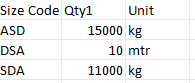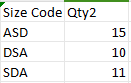# Linq Query For Table Comparison

Hello Folks ,
I have a linq query for table comparison but i need to change a condition that there is column in which unit is given ,if unit is Kg divide qty1 by 1000 and compare

dt_data.AsEnumerable.All(Function(x)Not String.IsNullOrWhiteSpace(x(“Size code”).ToString) AndAlso CDbl(x(“qty2”)) <= CDbl(x(“qty1”)) )

Could you maybe provide us with a Sample data that you are working on ? As there are some data adherences/format need to be cleared.

This way we would be able to suggest appropriate solutions faster.

Try This

dt_data.AsEnumerable.All(Function(x) Not String.IsNullOrWhiteSpace(x(“Size code”).ToString) AndAlso _
CDbl(x(“qty2”)) <= If(x(“Unit”).ToString.Trim.ToUpper = “KG”, CDbl(x(“qty1”)) / 1000, CDbl(x(“qty1”))))

Hi,
you want to modify a LINQ query to include a condition where you compare `qty2` to `qty1` after adjusting `qty1` based on the unit. If the unit is “Kg,” you need to divide `qty1` by 1000 before making the comparison. you can try below LINQ query:

``````dt_data.AsEnumerable.All(Function(x) Not String.IsNullOrWhiteSpace(x("Size code").ToString) AndAlso
CDbl(x("qty2")) <= If(x("Unit").ToString = "Kg", CDbl(x("qty1")) / 1000, CDbl(x("qty1"))))
``````

In this query, I’ve added a check for the unit (`x("Unit").ToString = "Kg"`) and adjusted `qty1` accordingly (`CDbl(x("qty1")) / 1000`). The `If` statement is used to conditionally divide `qty1` by 1000 if the unit is “Kg”; otherwise, it leaves `qty1` unchanged. The comparison is then made between `qty2` and the adjusted `qty1`.

DT1DT2i have joined The Table And Compared The Data

Thanks For The Solution

Cheers

This topic was automatically closed 3 days after the last reply. New replies are no longer allowed.